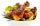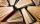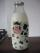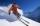# Three friends

Peter is eight times older than Rado, Rado is 3 times younger than Joseph. Together they have 120 years. Determine how many are each of them.

Result

P =  80
R =  10
J =  30

#### Solution:

P=8R
R = J/3
P+R+J= 120

P-8R = 0
J-3R = 0
J+P+R = 120

J = 30
P = 80
R = 10

Calculated by our linear equations calculator.

Leave us a comment of example and its solution (i.e. if it is still somewhat unclear...):

Showing 0 comments:Be the first to comment!#### To solve this example are needed these knowledge from mathematics:

Do you have a linear equation or system of equations and looking for its solution? Or do you have quadratic equation?

## Next similar examples:

1. SummerjobThree students participated in the summerjob. Altogether they earn 1780, -. Peter got a third less than John and Paul got 100,- more than Peter. How much got every one of them?
2. Father and sonWhen I was 11, my father was 35 years old. Today, my father has three times more years than me. How old is she?
3. Three daysDuring the three days sold in stationery 1490 workbooks. The first day sold about workbooks more than third day. The second day 190 workbooks sold less than third day. How many workbooks sold during each day?
4. SchoolsThree schools are attended by 678 pupils. To the first attend 21 students more and to the third 108 fewer students than to second school. How many students attend the schools?
5. Three figures - numbersThe sum of three numbers, if each is 10% larger than the previous one, is 662. Determine the figures.
6. Dining roomThe dining room has 11 tables (six and eight seats). In total there are 78 seats in the dining room. How many are six-and eight-seat tables?
7. Mom and daughterMother is 39 years old. Her daughter is 15 years. For many years will mother be four times older than the daughter?
8. The largerThe larger of two numbers is nine more than four times the smaller number. The sum of the two numbers is fifty-nine. Find the two numbers.
9. Football seasonDalibor and Adam together scored 97 goals in the season. Adam scored 9 goals more than Dalibor. How many goals scored each?
10. Farm2The farm bred turkeys, geese, hens and chickens. Everything is 400. Turkeys and geese a hen are 150. No hen has more than one chicken, but some hens has no chick. Half of them is also a quarter of the chickens. When the turkey subtracting 15, we get 75. Ho
11. Pages of bookLenka calculated that if every day to read 16 pages of the book, read a book a day earlier than had read only 14 pages a day. How many pages has this book?
12. The professor's birthdayProfessor of mathematics had 57 birthdays. The director congratulated him. The professor asked the director: "And how old are you?" The director replied: "I'm exactly twice as many years than you were when I was old as to you today." How old is the direc
13. Fifth of the numberThe fifth of the number is by 24 less than that number. What is the number?
14. TipplerBottle with cork cost 8.8 Eur. The bottle is 0.8 euros more expensive than cork. How much is a bottle and the cork?
15. Magic numberThe number 135 split to two addends so that one addend was 30 greater than 2/5 the addend.
16. Ski lessonsOn the ski course leaves 80 pupils. They live in triple and quadruple rooms. Count if rooms is the 23. How many are triple and quadruple rooms?
17. TreesAlong the road were planted 250 trees of two types. Cherry for 60 CZK apiece and apple 50 CZK apiece. The entire plantation cost 12,800 CZK. How many was cherries and apples?